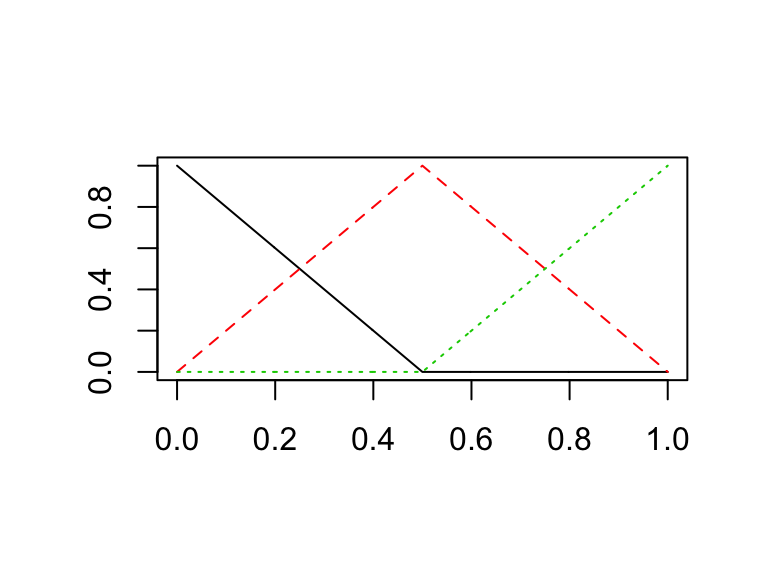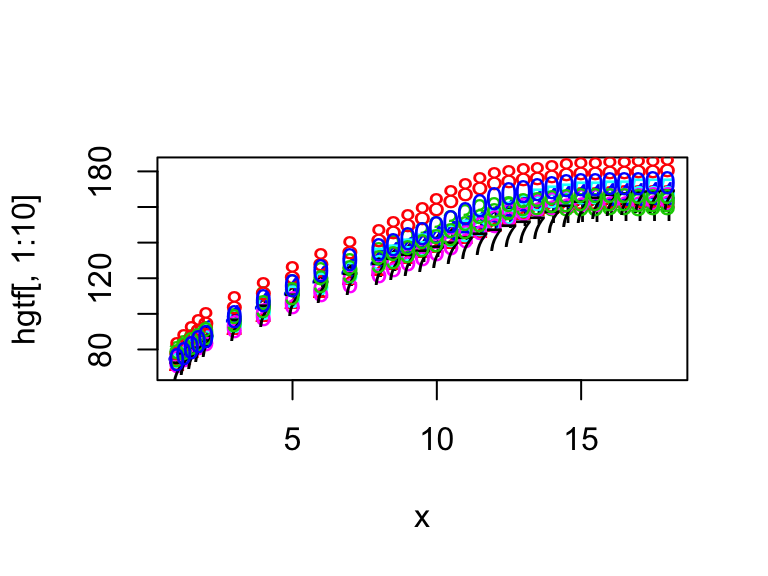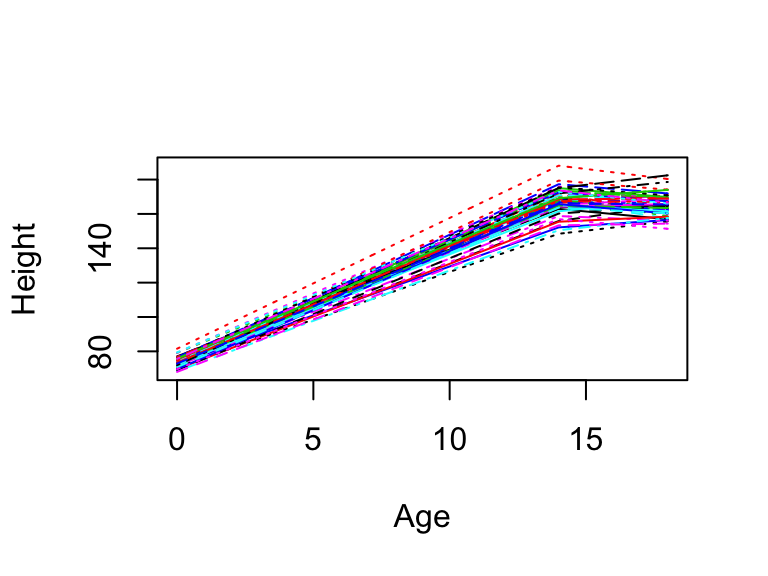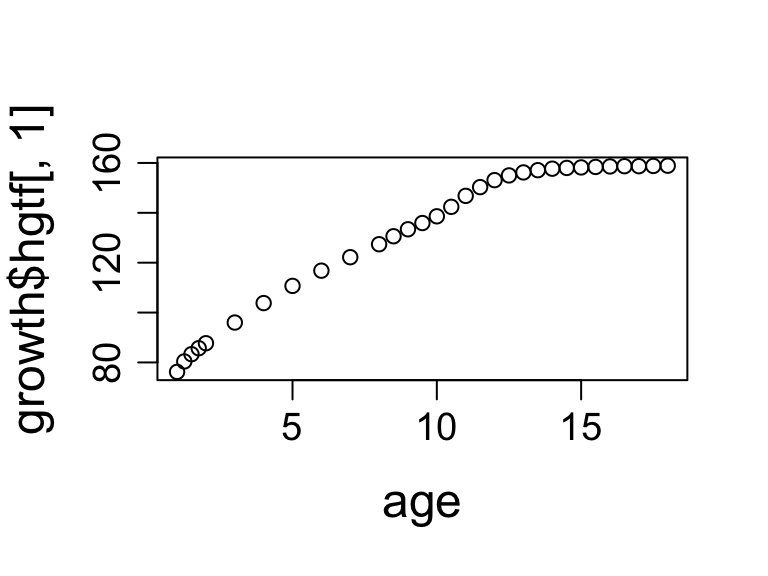### What Is Functional Data Analysis (FDA)?

• Analysis of information on curves or functions
• Examples of questions of interest: – What are the many ways in which different curves (e.g., growth curves of height, handwriting, lip movements) vary from one replication to another? – In what ways do they show regularity?
• Uses derivatives of the curves
• The mathematical descriptions of curves (including curves of derivatives) take on a very flexible form.
• Extensive repertoire of tools for smoothing data and estimating (smoothed or unsmoothed) derivatives, which can then be used in other statistical analyses.
• Procedures for estimating the ways in which, and the points at which the relationships among derivatives may deviate from constancy (i.e., may be time-varying).
• For more details on the approach illustrated here see: –Chow, S-M., Bendezú, J. J., Cole, P. M., & Ram, N. (2016). A comparison of two-Stage approaches for fitting nonlinear ordinary differential equation (ODE) models with mixed effects. , 154-184. Doi: 10.1080/00273171.2015.1123138.

First, let’s install the package .

## Polygonal (piecewise linear) basis functions

• Here, we will use the function `create.polygonal basis` to create a basis of (piecewise linear) spline coefficients over the interval [0,1] with break points at 0, 0.5, and 1.
• Adding dropind=1 to create.polygonal.basis drops the last basis function with break point at 1.
• The # of basis functions = the order of the system of basis functions, and it is one higher than the highest power (for the intercept).
``````library(fda)
polygonal = create.polygonal.basis(argvals=seq(0,1,0.5))
print(polygonal)``````
``````## \$call
## basisfd(type = type, rangeval = rangeval, nbasis = nbasis, params = argvals,
##
## \$type
##  "polyg"
##
## \$rangeval
##  0 1
##
## \$nbasis
##  3
##
## \$params
##  0.0 0.5 1.0
##
## \$dropind
## NULL
##
## NULL
##
## \$values
## list()
##
## \$basisvalues
## list()
##
## \$names
##  "polygon1" "polygon2" "polygon3"
##
## attr(,"class")
##  "basisfd"``````
``plot(polygonal)``## Polynomial/regression splines

-Polynomial splines are functions joined together smoothly at knots or breaks. The two outside knots define the range of the time intervals.

-Between two knots, a polynomial spline is a polynomial of fixed degree \(K_2\). But at an interior knot, two adjacent polynomials are required to match in the values of a fixed number of their derivatives, usually chosen to be \(K_2\)-1.

• Example: –A spline of degree 0 is a constant (a step function) that is discontinuous at knots. –A spline of degree 1 is a polygonal (i.e., piecewise linear function) that has the same level (0 derivative) at the interior knot points. – A spline of degree 2 is piecewise quadratic with first derivative that matches at the interior knot points.
• Degrees of freedom are equal to the number of coefficients to be estimated, that is, order (or highest power+1) + the number of interior knots.

# Berkeley Growth Example

``````#Load the Berkeley growth data set and extract height data
data(growth)
help('growth')

#The function "search()" allows us to check the packages we have loaded.
search()``````
``````##   ".GlobalEnv"        "package:fda"       "package:Matrix"
##   "package:splines"   "package:stats"     "package:graphics"
##   "package:grDevices" "package:utils"     "package:datasets"
``````#:: specifies that we want to use the matplot function from the fda package
with(growth, fda::matplot(age, hgtf[, 1:10], type="b"))````````````#Number of subjects you want to show in plots.
#Note that the fda library requires that each
#participant's data occupies a separate column.
#So all participants in the data set have the
#same number of measurement occasions (number
#of rows).
n = dim(growth\$hgtf)

#Ages
age =  c(seq(1, 2, 0.25), seq(3, 8, 1), seq(8.5, 18, 0.5))

#  Range of observations
rng =  c(1,18)

#Height of female participants
heightmat = growth\$hgtf

#Set up polygonal basis functions with a single interior knot at age 14
polygonal <- create.polygonal.basis(argvals=c(0,14,18))

#Create a functional parameter object, i.e., an "object"
#that carries all the functional parameters
growfdPar =  fdPar(polygonal)

#Creating a smoothed curve for the first participant using the
#FDA parameters set up in growfdPar.
#In this system of polygonal functions, the first segment has
#two coefficients to be estimated (intercept and linear slope);
#in the second segment, the intercept has to match the ending level
#of the first segment so only 1 more coefficient has to be estimated.
#So in total, we have df = 3 in this example.
heightfd0 = smooth.basis(age,growth\$hgtf[,1],growfdPar)
heightfd0\$df # 3``````
``##  3``
``````#You can also smooth all participants' data all at once -
#in other words, the same smoothing parameter is applied to all
#participants.
heightfd = smooth.basis(age,heightmat,growfdPar)

plot(heightfd,ylab="Height",xlab="Age")````````##  "done"``
``````#Plot one by one - not run
#par(cex.lab=1.5,cex.axis=1.2,mgp=c(2.75,.75,0))
#plotfit.fdSmooth(heightmat,age,heightfd,ylab="Height",xlab="Age")

#Use measurement ages as the knots
polygonal <- create.polygonal.basis(age)
growfdPar =  fdPar(polygonal)
heightfd = smooth.basis(age,heightmat,growfdPar)

#Setting graphical parameters
par(cex.lab=1.5,cex.axis=1.2,mgp=c(2.75,.75,0))
#Plot the first participant's growth curve
plot(age, growth\$hgtf[,1], type="p")````````````#Helpful output to extract
heightfd.fd = heightfd\$fd # functional data object
height.df = heightfd\$df #degrees of freedom
#generalized cross-validation index - lower indicates better fit
height.gcv = heightfd\$gcv
yhat = eval.fd(age,heightfd.fd,0) #Get smoothed level curves
Dxhat  = eval.fd(age, heightfd.fd, 1) #Get first derivatives
D2xhat = eval.fd(evalarg=age, fdobj=heightfd.fd, 2) #Get second derivatives``````

## Generate Data Using a Dampled Oscillator Model

``````#Library to obtain numerical solutions for ODEs for data generation
library('deSolve')
source('SelectFunctions.r')
# Simulate a sample of oscillators.
totalPersons <- 30 #Total number of participants
totalSamples <- 60 #Total number of time points
lastT <- 30
deltaT <- lastT / totalSamples
theTimes  <- seq(0, lastT, length=totalSamples)  # the measured time points
parms <- c(-0.5, -0.05) #eta, zeta
dataMatrix <- matrix(NA, nrow=totalSamples, ncol=totalPersons)
trueX <- matrix(NA, nrow=totalSamples, ncol=totalPersons)
truedX <- matrix(NA, nrow=totalSamples, ncol=totalPersons)

#Structuring the data in similar format as the growth data.
#Thus, each person has one column of data.
for (id in 1:totalPersons) {
parmsi  <- c(parms, parms + rnorm(1,mean=0,sd=.02))
xstart <- c(x=runif(1,min=-9,max=9), y=rnorm(1,mean=0,sd=2))
out1 <- as.data.frame(lsoda(xstart, theTimes, Osc, parmsi))
dataMatrix[,id] <- out1\$x + rnorm(length(out1\$x),0,.1)
trueX[,id] = out1\$x
truedX[,id] = out1\$y
}``````

## Read in Simulated Data and Compute Smoothed Derivative Estimates

Up till this point, we have generated data one particular ordinary differential equation (ODE) model - the damped oscillator model with individual differences in the zeta parameter only, with additive measurement noise. Now we will calculate derivative estimates and use them in a linear mixed effects model.

``````library('fda')
source('SelectFunctions.r')
totalPersons <- 30 #Total number of participants
totalSamples <- 60 #Total number of time points
lastT <- 30
deltaT <- lastT / totalSamples
theTimes  <- seq(0, lastT, length=totalSamples)  # the measured time points
rng =  range(theTimes)
#  We use b-spline basis functions of order 6
# 2 order higher than the highest derivative
# order used (4 in the penalty, see below)

#  Knots are positioned at the observation times.
norder =  6
nt   =  length(theTimes)
nbasis =  nt + norder - 2
oscbasis = create.bspline.basis(rng, nbasis,norder, theTimes)

#Penalizes 4th derivative as we are hoping to obtain smoothed
# second derivative estimates
roughPenaltyMax    =  4
lambda = .1  # a positive smoothing parameter: larger -> more smoothing
OscfdPar =  fdPar(oscbasis, roughPenaltyMax, lambda)

loglamout = plotGCVRMSE.fd(-6, -1, 0.25, theTimes, dataMatrix,
OscfdPar, FALSE)

#Based on the plot I decided to set lambda to 10^(-2)
OscfdPar =  fdPar(oscbasis, roughPenaltyMax, 10^(-2))

#Performing smoothing
oscfd = smooth.basis(theTimes,dataMatrix,OscfdPar)